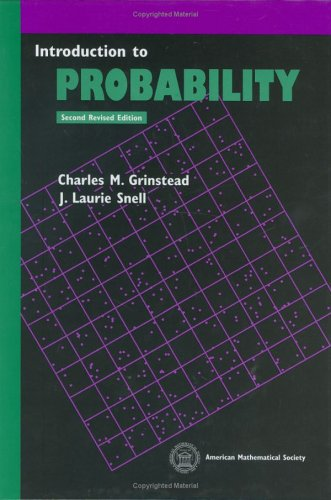Back to main page

## Introduction to ProbabilityThese are my proposed solutions. If you find any mistakes, please let me know.
A lot of simulation exercises. These were done in R.
Skipping chapters 10 and out. Only interesting topic is Markov chains, but there are better books for that.

#### Chapter 1 - Discrete Probability Distributions

1.1 Simulation of Discrete Probabilities
1.2 Discrete Probability Distributions

#### Chapter 2 - Continuous Probability Densities

2.1 Simulation of Continuous Probabilities
2.2 Continuous Density Functions

#### Chapter 3 - Combinatorics

3.1 Permutations
3.2 Combinations
3.3 [Skipped] Card Shuffling

#### Chapter 4 - Conditional Probability

4.1 Discrete Conditional Probability
4.2 Continuous Conditional Probability

#### Chapter 5 - Distributions and Densities

5.1 Important Distributions
5.2 Important Densities

#### Chapter 6 - Expected Value and Variance

6.1 Expected Value
6.2 Variance of Discrete Random Variables
6.3 Continuous Random Variables

#### Chapter 7 - Sums of Random Variables

7.1 Sums of Discrete Random Variables
7.2 Sums of Continuous Random Variables

#### Chapter 8 - Law of Large Numbers

8.1 Discrete Random Variables
8.2 Continuous Random Variables

#### Chapter 9 - Central Limit Theorem

9.1 Bernoulli Trials
9.2 Discrete Independent Trials
9.3 Continuous Independent Trials

#### Chapter 10 - Generating Functions

10.1 [Skipped] Discrete Distributions
10.2 [Skipped] Branching Processes
10.3 [Skipped] Continuous Densities

#### Chapter 11 - Markov Chains

11.1 [Skipped] Introduction
11.2 [Skipped] Absorbing Markov Chains
11.3 [Skipped] Ergodic Markov Chains
11.4 [Skipped] Fundamental Limit Theorem
11.5 [Skipped] Mean First Passage Time

#### Chapter 12 - Random Walks

12.1 [Skipped] Random Walks in Euclidean Space
12.2 [Skipped] Gambler’s Ruin
12.3 [Skipped] Arc Sine Laws